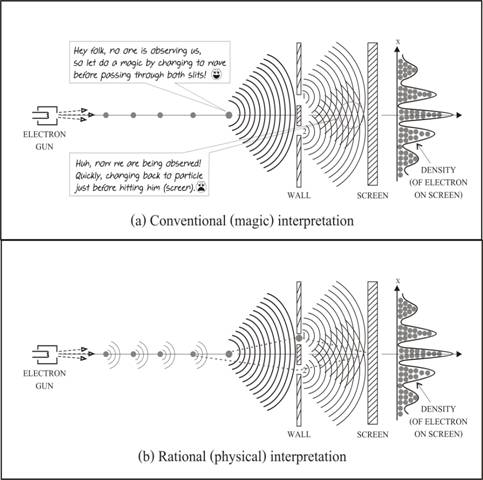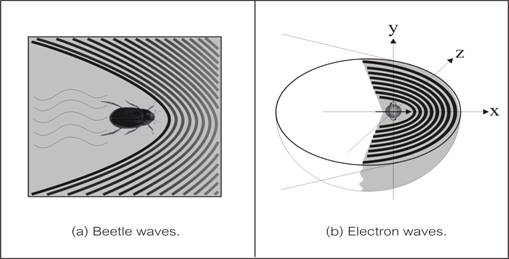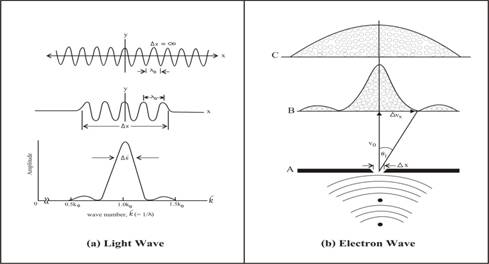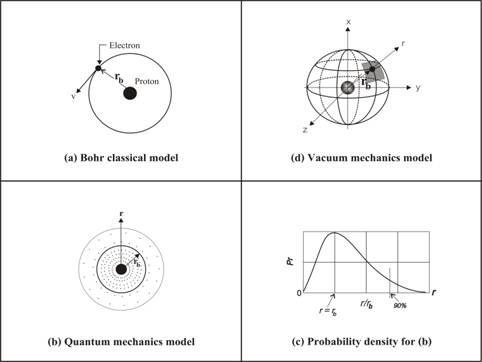# What is electron wave - particle duality, could we understand it?

Nimit Theeraleekul B. Eng. (Communication)

(1 October 2011)

In classical physics, we are familiar with electron which was classified as particle. And in our daily life, its application (which we used to) is a cathode ray tube of TV monitor. But in quantum physics, electron can also act as wave, and an application (which we are not so familiar) is electron microscope.

Now the problem is that no one could understand why and how electron can act as both particle and wave as show in the two-slit interference experiment (a). Armed with a new concept of vacuum medium, it is not difficult to explain its mechanism (b)! Here is a short summary, detail could be found in the article “Completed quantum mechanical theory” present in www.vacuum-mechanics.com .

1) First let us start with the diagram fig.1 (a) which is the famous two-slit interference experiment done by physicists. In conventional interpretation, electron particle converts itself into waves, passing through both slits and then the two part of waves collapse back into a single particle on screen. But the problem is how and why electrons could do the magic mentioned. This is the crucial problem in quantum mechanics called waves collapse interpretation!Figure 1 Two-slit electrons interference

Note that even though that the interference pattern which occurred on screen comes from stream of electrons, but it is not the interference between two electrons; instead it comes from one single electron which interferes with itself! Of course, one electron cannot create interference pattern, only a large amount of them could do. And this principle is also applied to photon, and which means that each photon will interfere with itself forming interference pattern!

2) Before solving the waves collapse interpretation problem as shown in diagram fig.1 (b), let us first point out that electron particle act as wave only when it is moving (we have never seen its wave property when it stay at rest), and there is no explanation about this! The root problem is because the concept of empty vacuum space in quantum mechanics. But, we have learned (from early article “Dark energy is in front of your eyes”) that vacuum space is not empty, instead it is vacuum medium space! Then it is easy to visualize and understand how electrons can do the magic as follow.Figure 2 Matter wave and particle wave

3) First it is easy to understand electron particle wave by using method of analogy with “surface water waves” created by a moving water beetle as show in the diagram of fig. 2(a). Normally, we are familiar with ship waves which occurred behind the moving ship, but for a small moving object such as a water beetle, the generated waves occur in front around the moving beetle!

In the same way, when an electron particle moving through vacuum medium, it will rotate while disturbing and creating standing waves around the electron as shown in fig. 2 (b). Note that the diagram shown is a three dimensions which was cut horizontally in x – z plane to show only the lower half part.

Anyway, there are two different points between beetle wave and electron wave; first, the former is a 2-dimension wave, while the latter is a 3-dimension wave. Second, beetle wave is a vertical transverse of water surface wave, while electron wave is a rotational transverse wave in vacuum medium.

4) Now return to solving the waves collapse interpretation problem in diagram fig.1 (b); all the electron particles moving together with their own created waves. Some of electrons pass through slit 1, while others pass through slit 2 randomly. While each electron passes through slit 1 or 2, its co-moving waves will pass through both slit 1 and 2 at the same time. After passing through both slits, both the waves will interfere each other while moving toward screen. Because all electron particles must move together with their own created waves (they are co-existence), then it is not surprise that all electron particles are arranged and forming to be interference pattern!

Finally, now we could understand why and how electron can act as both particle and wave via vacuum mechanism, i.e. the mechanism of vacuum medium. Also we can explain and solve the problem of waves collapse interpretation (which is the foundation problem of quantum mechanics) via scientific method - not a magic anymore!

5) Someone who familiar with quantum mechanics would found that it is the most mystic   theory in which no one could understand (mentioned by R. Feynman, a Nobel Prize winner in physics). And it is not surprise that Einstein had said that “Quantum mechanics is not a complete theory even though it is correct”!

Another point of view comes from David Bohm, a famous physicist in quantum theory, who said that “Quantum mechanics should be called as quantum non-mechanics”. This is because even through the theory was called “quantum mechanics” which mean that it should be something as “mechanism” of quantum system, but it seems that there is no such thing which could regard as system of separate parts working together according to causal laws!

6) Could this means that there is no need any mechanism for quantum mechanics? Of course, it should not be that, otherwise it is a magic, not science! And by adding our new concept of vacuum mechanics into quantum mechanical theory, then we can improve it to a more complete theory (which should be called as “quantum vacuum mechanics”). From now on, we will show how to solve the problem in the conventional theory by using our new quantum vacuum mechanics!

7) First let us look at Schrödinger equation which is the most important equation which was used to find the solution for subatomic particle such as electron or atom. Anyway, it could not be done from fundamental law, but by “making use of some deep formal analogies between optics and classical particle mechanics”. The most interesting part is that it is a wave equation, but we do not know what the wave is!

Now we know that actually particle wave is vacuum medium wave. And we have learnt from CEMT that electromagnetic wave is also vacuum medium wave, but different aspects of the same one vacuum medium wave! So it is easy to derive Schrödinger wave equation in the same way as was done with electromagnetic wave, and then what we got is a more rational and understandable equation!

8) Next we will use our quantum vacuum mechanics theory to solve the problem in the conventional quantum mechanics theory, and the first example is the famous Heisenberg’s uncertainty principle, which state that “It is not possible to determine both the position and the momentum of a particle with unlimited precision”.

Once, R. P. Feynman has said that the uncertainty principle protects quantum mechanics”! Heisenberg recognized that if it were possible to measure the momentum and the position simultaneously with a greater accuracy, the quantum mechanics would collapse. The only question is why it is so, or what is its mechanism?

To answer the question, we will start with “wave packet” such as photon in classical mechanics, which is a portion of a continuous wave train as shown in the diagram of fig. 3 (a), then what we got is the relation between length x and wave number k is as (1) below.

∆x. ∆k ~ 1 …. (1),       ∆k = (2¶/h) ∆p …. (2),    ∆x. ∆p ≥ (2¶/h) ~ h …. (3)Figure 3 Mechanism of the uncertainty principle

If we reduce ∆x then ∆k is increase, and in the reverse when we reduce ∆k then ∆x is increase. This means that we cannot determine both the position and the wave number of a wave packet with unlimited precision! So this is the uncertainty principle in classical mechanics which is occurred due to the property of wave packet!

Now by apply the uncertainty relation of wave packet (1) to electron wave via “single-slit diffraction” experiment in the diagram 3 (b). Consider a beam of electrons of speed v pass through the slit and create diffraction pattern as shown. By relating the parameters; v, ∆v, ∆x and Ө. Together with de Broglie wavelength λ = h/p or (2), then what we get is ∆p.∆x ~ h. (3).

In conclusion, we could see that the uncertainty measurement of electron is due to the co-moving wave packet (of vacuum medium) created by the moving electrons. And the energy of the wave packet will guide the moving electrons forming to be the interference pattern. This is the mechanism of Heisenberg’s uncertainty principle!

It is interesting to note that if there were no vacuum medium, then the moving electrons cannot create electron wave. So the moving electrons will go straight pass though the slit direct to screen and pile up as a smooth curve (due to statistical randomness, not interference) as shown at the top of the diagram 3 (b). So in principle, we can make a measurement of electron with unlimited precision!

9) The second example is about the problem with Bohr classical model of hydrogen atom, which we are familiar and shown in diagram fig.4 (a). According to the model, electron is circulating around the center proton at Bohr radius for the stationary state, but it was criticized that atom is not stable because electron would radiate energy and spirals into the nucleus!

In quantum mechanics, what we got is a better model of hydrogen atom as shown in diagram 4 (b). It has shown the probability location of the electron around the proton nucleus for the ground state in atom, which was calculated using Schrödinger equation. And in diagram 4 (c) shown the radial probability density which is maximum where the radius r equal to Bohr radius. Anyway, even the model in quantum mechanics, there is still no explanation why electron can stay separate from the proton nucleus, and it still depends on Bohr assumption of the stationary state!Figure 4 Hydrogen atom models

Armed with quantum vacuum mechanics, we could use the model shown in diagram 4(d) for explanation whyelectron can stay separate from the proton nucleus and having the probability density” as in 4(a) and 4(b). The explanation is that normally electron can move freely (even at absolute zero degree temperature) in “Bohr shell” because it is the place where the Coulomb attraction force (between electron and proton) equal to the repelling force of the vacuum medium in between!

Finally we also knew that there is always a small vibration in any atom, except at absolute zero degree temperature! So any electron in any atom could not stay only within Bohr shell, instead it has the probability to move to other location out of Bohr shell when time goes on. Of course, the probability density of electrons is according to wave mechanics. So what we got now is a better explanation for hydrogen atom model which is more realistic and understandable!

10) The last example is the hot issue (problem) of quantum entanglement or correlation, in which one electron can affect another electron simultaneously, no matter how far they are separated. And it was claimed that this is the nature of the conventional quantum mechanics, but the problem is that they do not know how electron can do the magic!

Armed with our quantum vacuum, it is easy to show that this because of our ignorance in the mechanism of quantum mechanics, which is then, give us with misinterpretation in the theory as follow.

First, historically, quantum entanglement was criticized by Einstein, in which he called it as a spooky action at a distance! And this give rise to a debate between Einstein and Neil Bohr (a famous quantum physicist), via a thought-experiment on a two-particle system, called EPR paradox.

Second, because the conventional interpretation of quantum mechanics, in which measurement with one particle can affect the far away particle simultaneously, for Einstein this due to the incompleteness  of the theory! In Einstein opinion, there should have something (attribute) hidden, i.e. hidden variable, in which we cannot observe, that responsible for the mentioned “affect”.

Third, there are many experiments which tried to prove hidden variable concept by using a mathematical formula called Bell inequality. Anyway, most of the results seem to agree with quantum mechanics, not hidden variable. The reason is because the proposed hidden variable is not related to the property of the electron in the experiment!

Finally, based on the existence of vacuum medium in which electron wave was created by a rotating moving electron disturbs the surrounding vacuum medium! And by using electron wave as the hidden-variable mentioned, we would then found that the experimental result must agree with quantum mechanics. The reason is because what we have used as the hidden-variable is the mechanism of quantum mechanics, isn’t?

……………………………….